Latest Banking jobs   »

# Quantitative Aptitude Quiz For RBI Grade B Phase 1 2023 – 11th May

Directions (1-5): In each question two equations numbered (I) and (II) are given. You should solve both the equations and mark appropriate answer.
(a) If x=y or no relation can be established
(b) If x>y
(c) If x<y
(d) If x≥y
(e) If x≤y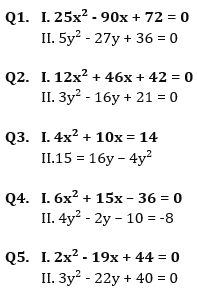Directions (6-10): Find the approximate value that will come in place of question mark(?) in the following questions.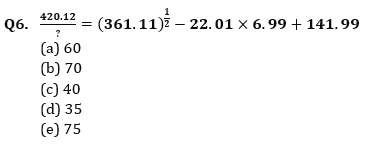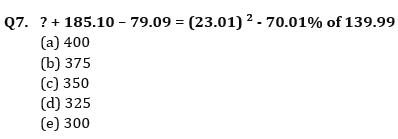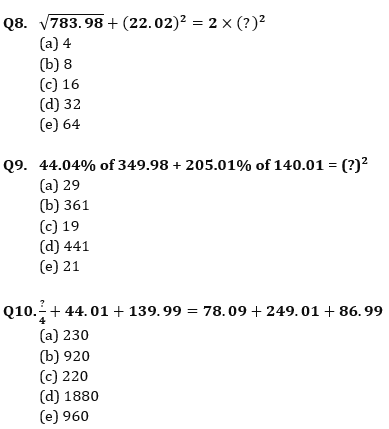Solutions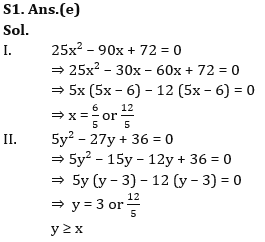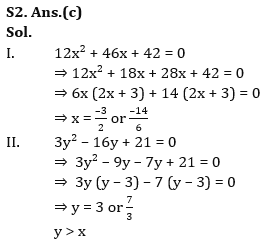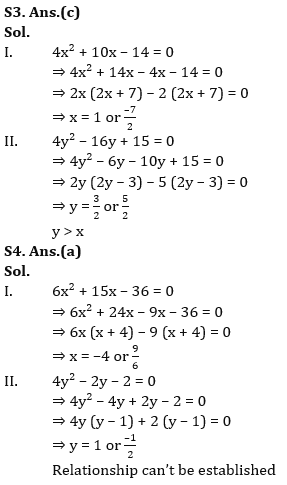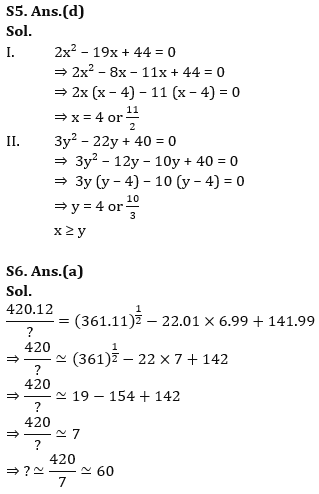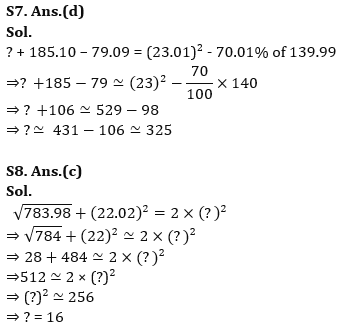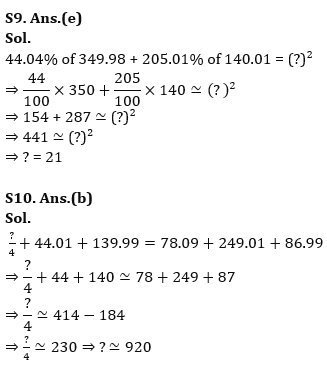.                                                                                                                                                                                                                                                                                                                                                                         .

## FAQs

### How many sections are there in the RBI Grade B Phase 1 Exam?

There are 4 sections in the RBI Grade B Phase 1 Exam i.e. English Language, General Awareness, Quantitative Aptitude & Reasoning.

#### Congratulations!Union Budget 2023-24: Free PDF